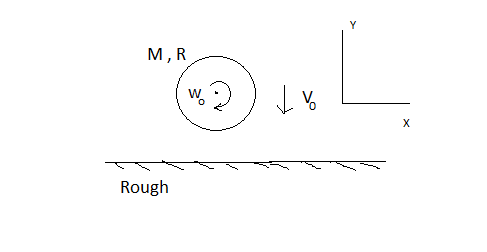# Inelastic Sphere Collision!A uniform rigid sphere having radius $R$ moving with velocity ${ v }_{ o }$ and angular velocity ${ \omega }_{ o } =\cfrac { { v }_{ o } }{ R }$ strikes a horizontal rough surface having coefficient of friction $\mu$ . If coefficient of restitution for collision is $e=1/2$.
Let angular velocity and Velocity of centre of sphere after immediately after the collision is in 'x' and 'y' direction will be expressed as :

\begin{aligned} { v }_{ x } &= \cfrac { a }{ b } \times \mu { v }_{ o }\\ { v }_{ y }&= \cfrac { c }{ d } \times { v }_{ o }\\ { { \omega } }^{ " }&= \cfrac { (\alpha -\beta \times \mu ) }{ \gamma R } \times { v }_{ o } \end{aligned}

Then find the value of :

$a + b + c + d + \alpha +\beta +\gamma.$

Details and assumptions

$\bullet$ gcd (a , b ) = 1

$\bullet$ gcd (c,d ) = 1

$\bullet$ gcd ($\alpha$ , $\beta$ ) = 1

$\bullet$ gcd ( $\gamma$ , $\beta$ ) = 1

$\bullet$ All are positive integers.

$\bullet$ Take clockwise direction as positive.

###### This is part of my set Deepanshu's Mechanics Blasts

Source : My Friend give me as challange , Unfortunately He is not on Brilliant .

×